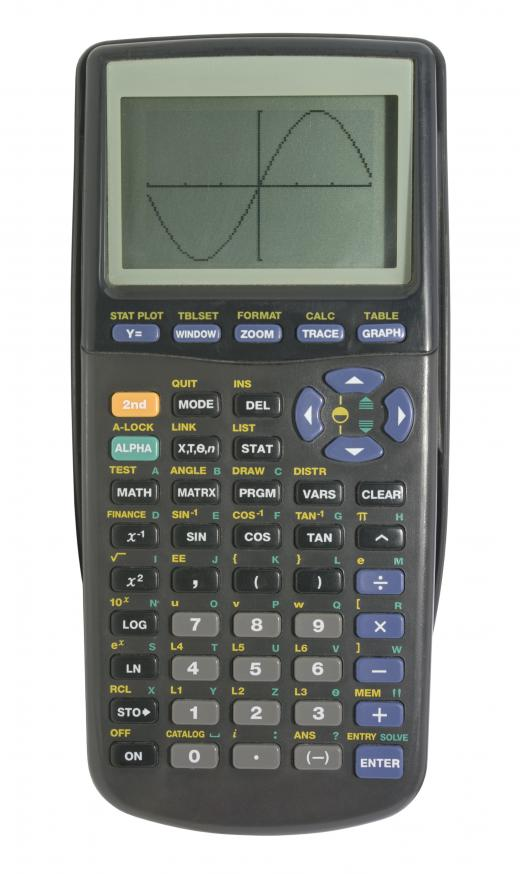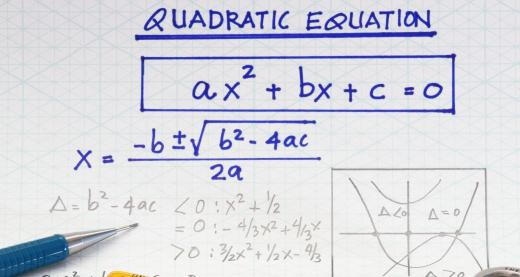# What is a Spline?

H.R. Childress
H.R. ChildressA graphing calculator may be used to calculate cubic spline interpolation.

A spline is a type of piecewise polynomial function. In mathematics, splines are often used in a type of interpolation known as spline interpolation. Spline curves are also used in computer graphics and computer-aided design (CAD) to approximate complex shapes.

Interpolation is used when there is a set of discrete data points and it is necessary to estimate other points of the same type of data from the given points. Polynomial interpolation is commonly used for small numbers of data points; this is a method that fits an n order polynomial function to n + 1 data points. When the number of points becomes larger, however, polynomial interpolations often do not fit the data well. In these cases, spline interpolation is often used instead.An interpolated y value on a quadratic spline is found by using the general quadratic equation.

While polynomial interpolation fits a curve through all the data points at once, spline interpolation approximates a curve between each proximate pair of data points and adds all the curves together to create the final approximation. This is why splines are piecewise functions rather than smooth curves. Commonly used spline interpolation techniques include linear, quadratic, and cubic interpolation.

Linear spline interpolation simply fits straight lines through each consecutive pair of data points. Each line section may have a similar or very different slope from the other section, depending on the distribution of the data. To find the y value on a Cartesian coordinate system for a given x value between two data points, the slope between the given points is multiplied by the distance between the x value for which the y value is desired and the x value for the point to its left. This number is then added to the y value to the left of the desired location to obtain the approximation for the y value between the two points.

Quadratic spline interpolation approximates the data between consecutive points by a quadratic polynomial. To find the coefficients of these quadratic equations, a number of methods for solving simultaneous equations may be applied. Linear algebra techniques or solving by use of computer software are some of the more common techniques used. An interpolated y value on a quadratic spline is found by using the general quadratic equation, y = a*x2 + b*x + c, with the a, b, and c coefficients previously determined.

Cubic spline interpolation uses a cubic, or third order, polynomial function to approximate the data between consecutive points. This type of spline is usually calculated using computer software or a graphing calculator. A special type of cubic spline interpolation, called clamped or complete spline interpolation, uses slopes given at the ends of the curve to help compute the function.